Perpendicular lines show up all over the place in geometry.  They also have many applications in real life – but before you can use them, you need to understand them!

So, what do you need to know about perpendicular lines?  Two perpendicular lines intersect each other at a 90 degree angle, and they have opposite reciprocal slopes (m and -1/m). Perpendicular lines meet at exactly one point, and they are never parallel. A line cannot be perpendicular to itself. The dot product of two perpendicular vectors is always zero.

Of course, we also see perpendicular lines in certain geometric shapes, such as right triangles.  The resulting right triangles are helpful for the geometry used in construction, graphics, and other applications.

Let’s get started.

## What Are Perpendicular Lines?

Two lines are perpendicular if they meet (intersect) at a 90 degree angle.  For example, the two lines pictured below are perpendicular.

Two perpendicular lines will have opposite reciprocal slopes.  For example, the line y = (3/2)x is perpendicular to the line y = (-2/3)x.  You can see this in the picture below.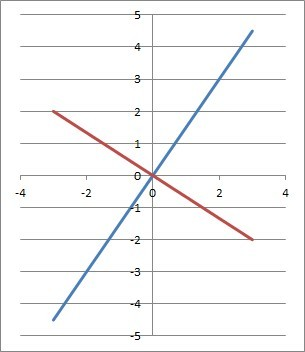The lines y = (3/2)x (blue line) and y = (-2/3)x (red line) are perpendicular, since they have opposite reciprocal slopes.

We can also discuss perpendicular rays, sides, or line segments.  They have the same meaning: the two rays, sides, or line segments meet at 90 degree angles.

Any geometric shape with two perpendicular sides has a 90 degree angle.  For example, the right triangle below has two perpendicular sides (the legs of the triangle).

### Can Perpendicular Lines Intersect?

Perpendicular lines can intersect – in fact, two lines must intersect to be perpendicular.  This point of intersection is necessary to give us a point of reference for the 90 degree angles formed where the perpendicular lines meet.

#### Where Do Two Perpendicular Lines Intersect?

Two perpendicular lines intersect at exactly one point, where we see 90 degree angles formed.  Given the equation of two perpendicular lines, we can find their point of intersection as follows.

Let’s say the two lines are given by

• y1 = mx + b1
• y2 = (-1/m)x + b2

where m is not zero.

We know that these two lines are perpendicular because they have opposite reciprocal slopes: m and -1/m.

To find out where they meet, we set the “y” parts equal and solve for x:

• y1 = y2
• mx + b1 = (-1/m)x + b2
• mx + (1/m)x = b2– b1
• (m + (1/m))x = b2– b1
• ((m2 + 1)/m)x = b2– b1
• (m2 + 1)x = m(b2– b1)
• x = m(b2– b1) / (m2 + 1)

Note: if m = 0, we have a pair of lines: one horizontal, one vertical.  For any vertical line of the form x = c, a horizontal line y = d is perpendicular.

Let’s look at an example with some numbers.

##### Example: Finding The Intersection Of Two Perpendicular Lines

Let’s say the two perpendicular lines are given by

• y1 = 2x + 12
• y2 = (-1/2)x – 3

Here, we have m = 2, b1 = 12, and b2 = -3.  Using the formula we found before, we can solve for the value of x where these lines intersect:

• x = m(b2– b1) / (m2 + 1)
• x = 2(-3– (12)) / (22 + 1)
• x = 2(-15) / (5)
• x = -6

Now that we know the value of x, we can substitute into either line equation to find y.  We’ll use y1:

• y1 = 2x + 12
• y1 = 2(-6) + 12
• y1 = 0

So, the point of intersection of these two perpendicular lines is (-6, 0).  In this case, the intersection point is on the x-axis, but this is not always the case.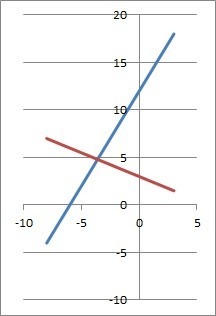The lines y = 2x + 12 (blue line) and y = (-1/2)x + 3 (red line) are perpendicular, since they have opposite reciprocal slopes.

### Can Perpendicular Lines Be Parallel?

Perpendicular lines cannot be parallel.  There are two ways to see this.

One is to remember that perpendicular lines must intersect, but parallel lines never intersect.  So, a pair of lines can never be both perpendicular and parallel: it is one or the other or neither.

Another way is to remember that perpendicular lines have opposite reciprocal slopes, but parallel lines have the same slopes.  If the slope of one of the lines is m, then the slope of a perpendicular line is -1/m.

We can show that there is no case where m = -1/m, since:

• m = -1/m
• m2 = -1
• m = √-1

This suggests an imaginary slope, which is not possible for a line y = mx + b with real x and y values.

So, perpendicular lines cannot be parallel.  Similarly, parallel lines cannot be perpendicular.

### Can Perpendicular Lines Be Coplanar?

Perpendicular lines are always coplanar, since they must intersect, and they do so in a particular plane.

#### Example: Perpendicular Lines Are Coplanar

Consider the two perpendicular lines x = -3 and y = 1.  In 2-dimensional space, they are perpendicular, since they meet at a 90 degree angle at the point (-3, 1) – we can see this in the graph below.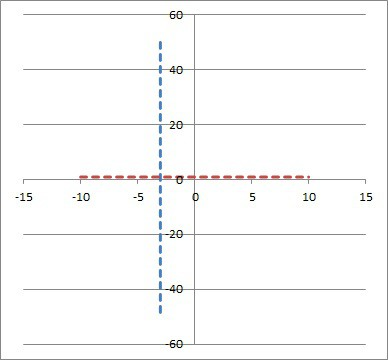The vertical line x = -3 (blue line) and the horizontal line y = 1 (red line) are perpendicular, since they meet at a 90 degree angle.

If we extend this concept to three dimensions by adding a z-axis, we can say that the lines x = 1 and y = 2 both exist in the plane z = 0.  In fact, they intersect and are perpendicular within in this plane.

### Can A Line Be Perpendicular To Itself?

A line cannot be perpendicular to itself.  A line can only have one slope, and the slope cannot be the opposite reciprocal of itself.

That is, if the slope of the line is m, there is no real number such that m = -1/m.  As we showed earlier, this suggests an imaginary slope, which is impossible:

• m = -1/m
• m2 = -1
• m = √-1

### What Is The Dot Product Of Perpendicular Vectors?

The dot product of two perpendicular vectors is zero.  We can prove this by using one of the formulas for the dot product.

If a and b are vectors with an angle of C between them, then their dot product is given by the formula:

• a•b = ||a||*||b||*cos(C)

where ||a|| is the magnitude of vector a and ||b|| is the magnitude of vector b.

If a and b are perpendicular vectors, then the angle C between them is 90 degrees (by definition of perpendicular).

Substituting C = 90 into the dot product formula, we get:

• a•b = ||a||*||b||*cos(90)
• a•b = ||a||*||b||*0  [since cos(90) = 0]
• a•b = 0  [a product of any number with zero is zero]

So, the dot product of vectors a and b is zero is the angle between them is 90 degrees.

### Does A Trapezoid Have Perpendicular Sides?

Some trapezoids may have perpendicular sides, but not every trapezoid does.  Remember that a trapezoid is defined as a quadrilateral (four-sided shape) with at least one pair of parallel sides.

If both pairs of sides in a trapezoid are parallel, then it only has perpendicular sides if the shape is actually a rectangle (which includes the more specialized case a square).  In this case, there are four 90 degree angles, as seen in the image below.A rectangle is a specific type of trapezoid with two pairs of parallel sides and four 90 degree angles. Each pair of adjacent sides is perpendicular.

If only one pair of sides in a trapezoid is parallel, then the trapezoid may have perpendicular sides if two of the angles are 90 degrees.  You can see an example of this in the image below.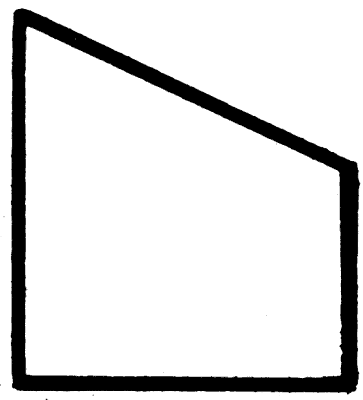This trapezoid has only one pair of parallel sides (the left and right sides). The bottom side is perpendicular to the left and right sides, which means we have two 90 degree angles. The top two angles are supplementary, adding up to 180 degrees.

We can also have a trapezoid with no 90 degree angles at all, as seen in the image below.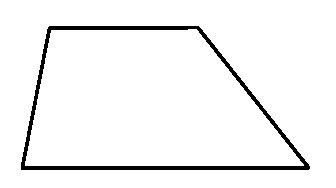This trapezoid has no right angles, and thus no perpendicular sides. The top and bottom sides are parallel.

### Does A Rhombus Have Perpendicular Sides?

In some cases, a rhombus has perpendicular sides, but not all of them do.  Remember that a rhombus is defined as a quadrilateral with four sides of the same length.

Any square counts as a rhombus, since all four sides have the same length.  However, it has the extra condition that the angles are all 90 degrees, meaning that any pair of adjacent sides is perpendicular.A square is a specific type of rhombus with four 90 degree angles. As such, any pair of sides is perpendicular.

Any rhombus that is not a square has no 90 degree angles, and thus no perpendicular sides.  We can prove this as follows:

Imagine that we have a rhombus that is not a square, and the angles are w, x, y, and z.

Also assume that w and y are opposite angles, so w = y.  Then x and z are opposite angles, so x = z.

Let’s say that one of the angles is 90 degrees.  If w = 90, then its opposite angle y must also be 90 degrees.  The reason is that for any rhombus, opposite angles are equal.

The four interior angles of any four-sided shape add up to 360, so we have:

• w + x + y + z = 360
• 90 + x + 90 + z = 360  [since w and y are both 90 degrees]
• z + z = 180  [x = z, since x and z are opposite angle in a rhombus]
• 2z = 180
• z = 90

Since z = 90, then x = 90 also, because z = x.

So, all four angles in the rhombus are 90 degrees, which is impossible (we said that the rhombus was not a square).

## Conclusion

Now you know about perpendicular lines and how they relate to parallel lines, dot products, and some of the geometric shapes.